# Reidemeister torsion

Jump to: navigation, search

de Rham torsion, Franz torsion

An invariant which allows one to distinguish many structures in differential topology, for example knots and smooth structures on manifolds, particularly on lens spaces. Reidemeister torsion was first introduced by K. Reidemeister (see ) while studying three-dimensional lenses, the generalization for-dimensional lenses was obtained independently in  and .

Letbe a free complex of left-modules, whereis an associative ring with a unit element. Further, letbe a matrix representation of, i.e. a homomorphism frominto the ring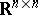of all real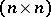-matrices. Let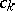be distinguished bases in the modules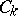of the complex, and let the complex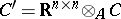of-modules be acyclic; then the Whitehead torsion is defined as, whereis the multiplicative group of the field of real numbers. The numberis called the Reidemeister torsion of the complex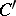, and also the real Reidemeister torsion.

The usefulness of transforming the Whitehead torsion into the Reidemeister torsion is based on Bass' theorem . Ifis a finite group, then the elementhas finite order if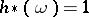for any representation, whereis the Reidemeister torsion induced by the element.